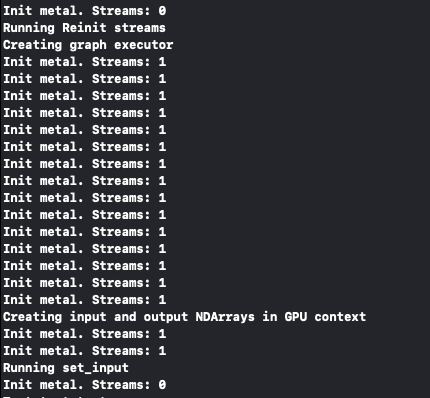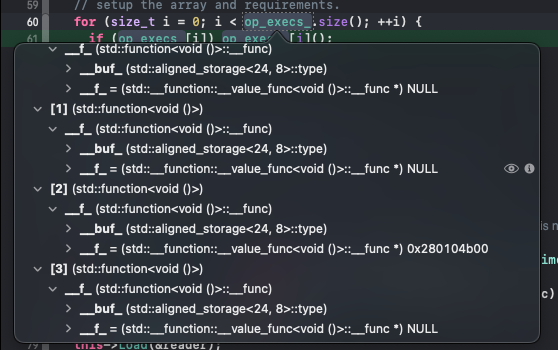# TVM iOS Metal Failing to Sync

Hello again, I am trying to create a simple proof of concept of loading a model, running it, and retrieving the results using metal on an iOS device, in this case an ipad 6th gen with iOS 15.1, model number MR7D2LL/A. This is being compiled and run on the iPad using Xcode 13.1.
It seems to run well, loading the compiled model and creating the graph executor on the metal device, until a copy or sync operation calls the `CastStreamOrGetCurrent` function in the `metal_device_api.mm` file. When this function is called, the program crashes with a `exec_bad_access` error.

Looking into what is returned from the `MetalThreadEntry::ThreadLocal()` call, it is apparent that the stream array is empty.

Here is how I am compiling the model in python (inspired by the ios_rpc apps example):

``````from tvm.contrib import xcode

shape_dict = {"data": (1, 3, 512, 512)}
arch = "arm64"
sdk = "iphoneos"

@tvm.register_func("tvm_callback_metal_compile")
def compile_metal(src):
return xcode.compile_metal(src, sdk=sdk)

builder = xcode.create_dylib
mod, params = relay.frontend.from_onnx(model, shape_dict)
with tvm.transform.PassContext(opt_level=0):
target = tvm.target.Target(target="metal", host="llvm -mtriple=%-apple-ios -link-params" % arch)
compiled = relay.build(mod, target=target, params=params)

file = open("./mod_metal.json", "w")
file.write(compiled.get_executor_config())
file.close()

compiled.get_lib().export_library(compiled_model,fcompile=builder, arch=arch, sdk=sdk)
``````

And here is the stripped down C++ that I am currently using to execute the model on metal (type declarations are elsewhere):

``````    std::ifstream model_json_in("mod_metal.json", std::ios::in);
std::string json_data{(std::istreambuf_iterator<char>(model_json_in)),std::istreambuf_iterator<char>()};
model_json_in.close();
model_json = json_data;

ctx = {kDLMetal, 0};

mod_factory = (*tvm::runtime::Registry::Get("tvm.graph_executor.create"));
executor = mod_factory(json_data, mod_syslib, (int64_t)ctx.device_type, (int64_t)ctx.device_id);

set_input = executor.GetFunction("set_input");
get_output = executor.GetFunction("get_output");
run = executor.GetFunction("run");

x = tvm::runtime::NDArray::Empty({1, 3, 512, 512}, DLDataType{kDLFloat, 32, 1}, ctx);
y = tvm::runtime::NDArray::Empty({1, 26, 15, 4096}, DLDataType{kDLFloat, 32, 1}, ctx);

// Load data previously placed in tmpBuffer into x
TVMArrayCopyFromBytes((TVMArrayHandle)&x, reinterpret_cast<void *>(tmpBuffer), (size_t)(m_channels * m_width * m_height * sizeof(float)));

set_input("data", x); // Crashes here, in this case, when the CastStreamOrGetCurrent gets invoked
run();
get_output(0, y);
``````

Here is the stack trace upon crashing:

When I try copying the data into the gpu using this method, it crashes on this line, also when the `CastStreamOrGetCurrent` function is invoked

``````x.CopyFromBytes(reinterpret_cast<void *>(tmpBuffer), (size_t)(m_channels * m_width * m_height * sizeof(float)));
``````

And when I try running a manual sync, passing in `nullptr` for the stream, it also crashes when that function is called.

``````TVMSynchronize(ctx.device_type, ctx.device_id, nullptr);
``````

Does anyone have any hints as to where things may be going wrong? I’m not certain how the stream array is supposed to get populated, or what may be interfering with it.

Oh, just to specify, I am building the libtvm_runtime.dylib being compiled with the project using the method in the ios_rpc project, and I’ve compiled the local tvm installation with USE_METAL set to ON.

It looks like a thread is being created in ReinitializeStreams when the compiled model is being loaded, and according to the debugger, it does persist in the default_streams_ array, but not the ThreadLocal’s stream array by the time a sync is called.

For some reason, the `MetalWorkspace* MetalWorkspace::Global()` function is also being called when the graph_executor is being created, and when the set_input function is being called. Is this normal?

It looks like in the process of creating the graph executor with the gpu context, it calls the `MetalWorkspace::Init` function 15 times, calling the `MetalWorkspace::ReinitializeStreams()` the first time where it has zero streams on the `MetalThreadEntry::ThreadLocal()->stream` array when the model is loaded.Does this provide any insight?

Okay, this was partially my fault, it seems. I was actually creating the graph executor in the constructor of a class, and using it in one of its member functions that was called by an external source. Perhaps this resulted in a different thread being used when the set_input function was run? Is there no way to preserve access to the metal stream within the class?

Now, however, it is crashing on the `run()` step, giving another exec_bad_access error at the MetalWrappedFunc () operator function

Here is the stack trace of this error.

Does anyone have any ideas on what may be causing this?

Okay, it appears to be giving the error when trying to run the operator at index 2.

Taking a look at the operator array, the ones before and just after have a value function that is NULL, whereas 2 points to an address.Could there be an issue in how I am compiling the model, perhaps?

Edit: I’ve tried recompiling the model without the parameters linked flag, but the load_params function produces an error. New compilation in python (other steps untouched):

``````with tvm.transform.PassContext(opt_level=0):
target = tvm.target.Target(target="metal", host="llvm -mtriple=%-apple-ios" % arch)
compiled = relay.build(mod, target=target, params=params)
new_params = tvm.runtime.save_param_dict(compiled.get_params())
file = open("./mod_metal.params", "wb")
file.write(bytearray(new_params))
file.close()
file = open("./mod_metal.json", "w")
file.write(compiled.get_executor_config())
file.close()
compiled.get_lib().export_library(compiled_model,fcompile=builder, arch=arch, sdk=sdk)
``````

And the parameters are attempting to be loaded like so:

``````	std::ifstream params_in("mod_metal.params", std::ios::binary);
std::string params_data((std::istreambuf_iterator<char>(params_in)), std::istreambuf_iterator<char>());
params_in.close();
params_arr.data = params_data.c_str();
params_arr.size = params_data.length();
mod_factory = (*tvm::runtime::Registry::Get("tvm.graph_executor.create"));
executor = mod_factory(model_json, mod_syslib, (int64_t)ctx.device_type, (int64_t)ctx.device_id);
set_input = executor.GetFunction("set_input");
get_output = executor.GetFunction("get_output");
run = executor.GetFunction("run");